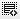## How to raise money using Debt?0
Hi Binny

How to raise money using debt

If you took \$20 million as debt to fund a \$200 million project. Assuming 10% interest on the debt and returns of \$500 million in 5 years. What is your ROI?

Total Investment = 200
Investment = 200-20= 180
Debt= 20
Interest = 10%
Years= 5
Return= 500

Debt + Interest for 5 years = 20 + 10 = 30

Returns - Debt payable = 500-30 =470

ROI= 470/180 = 261%

Please correct me if I am wrong.0

Kaushik, the ROI formula is (Return - Investment)/Investment = (470-180)/180 = 161%
The correct answer should be 161%. We will fix that answer choice.0

Oh ok ok ... Thank you

Click on thisicon to add code snippet.